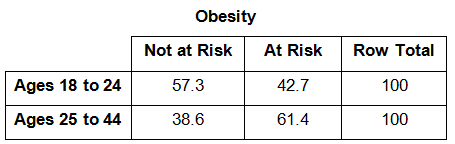# Statistics and Probability / Two Way Tables and Categorical Data

Activity 24 of 24
In this lesson, students will use two-way tables to investigate associations between two categorical variables.

## Planning and Resources

Objectives
Students recognize whether there is an association between categorical data using two-way tables and stacked bar graphs. They also determine conditional probabilities given information in a two-way table.

Vocabulary
two-way table
categorical data
relative frequency
conditional probability

Standard:

## Lesson Snapshot

#### Understanding

Conditional probabilities and/or stacked bar graphs can reveal potential associations among categorical variables.

### What to look for

Relative frequency is particularly important when comparing groups of different sample size.

### Sample Assessment

The table below describes risk factors for obesity associated with age.a) What do these number represent? Are they frequencies or relative frequencies or neither?

Answer: These numbers represent relative frequencies, but they are presented as percentages between 0 and 100 rather than as decimal numbers between 0 and 1.

b) Does there appear to be an association between age group and obesity risk?

Answer: There does appear to be ans association. The older group has a much higher percentage at risk than the younger group.

#### The Big Idea

Two-way tables of relative frequencies provide numerical evidence for whether or not there is an association between categorical variables, and the associated relative frequency graphs provide visual evidence.

### What are the students doing?

Students explore two-way tables and stacked bar graphs as tools for investigating whether or not there is an association between two categorical variables.

### What is the teacher doing?

Be sure students understand that the relative frequency graphs are particularly important when comparing categories where the total counts are different.